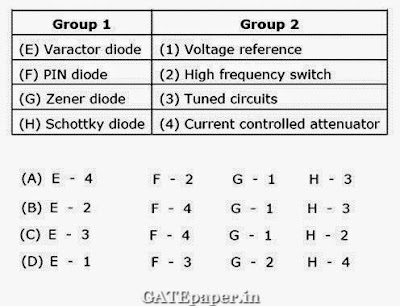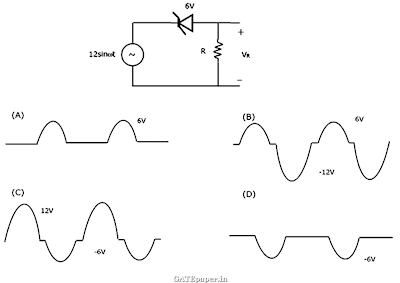### GATE 2006 ECE Video Solutions on EDC (Electronic Devices and Circuits)

1. The values of voltage across a tunnel diode (VD) corresponding to peak and valley currents are VP and VV respectively. The range of tunnel diode voltage VD for which the slope of its I-V characteristics is negative would be
a. VD < 0
b. 0 ≤ VD ≤ VP
c. VP ≤ VD ≤ VV
d. VD ≥ VV

2. The concentration of minority carriers in an extrinsic semiconductor under equilibrium is
a. Directly proportional to the doping concentration
b. Inversely proportional to the doping concentration
c. Directly proportional to the intrinsic concentration
d. Inversely proportional to the intrinsic concentration

3. Under low level injection assumption, the injected minority carrier current for an extrinsic semiconductor is essentially the
a. Diffusion current
b. Drift current
c. Recombination current
d. Induced current

4. The phenomenon known as “early effect” in a BJT refers to a reduction of the effective base width caused by
a. Electron – hole recombination at the base
b. The reverse biasing of the base collector junction
c. The forward biasing of emitter base junction
d. The early removal of stored base charge during saturation to cutoff switching

5. An N-channel depletion MOSFET has the following two points on its ID verses VGS curve are
(i) VGS = 0 at ID = 12 mA and
(ii) VGS = -6 volts at ID = 0 mA
Which of the following Q-points will give the highest Transconductance gain for small signals?
a. VGS = -6 volts
b. VGS = -3 volts
c. VGS = 0 volts
d. VGS = 3 volts

6. In the circuit shown below, the switch was connected to position 1 at t < 0, and at t = 0, it is changed to position 2. Assume that the diode has zero voltage drop and a storage time tS. For 0<t<tS, the voltage across the 1 KΩ resistor (VR) in volts is7. The majority carriers in an N-type semiconductor have an average drift velocity V in a direction perpendicular to a uniform magnetic field B. The electric field E induced due to hall effect acts in the direction
a. V x B
b. B x V
c. along V
d. opposite to V

8. Find the correct match between Group 1 and Group 2 :9. A heavily doped N-type semiconductor has the following data:
Hole – electron mobility ratio : 0.4
Doping concentration : 4.2 x 108 /m3
Intrinsic concentration : 1.5 x 104 /m3
The ratio of conductance of the N-type semiconductor to that of the intrinsic semiconductor of same material and at the same temperature is given by
a. 0.00005
b. 2000
c. 10000
d. 20000

10. For the circuit shown below, assume the zener diode is ideal with a breakdown voltage of 6 volts. The waveform observed across R is